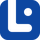# Articles related to "both"

### Either vs. Both

The difference between 'either' and 'both' is actually very easy to understand. Follow the article to fully grasp the differences and similarities.

### Each vs. Both

'Both' and 'each' have two different meanings which makes them easy to understand.

### Quantifiers

Quantifiers or Quantitatives are a type of determiners that are used with nouns. In this lesson, we will discover their uses, rules, and more in detail.

### Both vs. All

It is actually easy to understand the difference between these two words. In this lesson, we will learn more about them.

### Both vs. Each

'Each' and 'both' are easily confused by English learners. They are similar to each other. Follow the article to learn about their similarities and differences.

### Both vs. Together

Together or both? Where do we use them? Do they mean differently? These are the questions asked commonly by English learners. Let’s take a look at them.

### Distributives

Distributives are determiners that indicate divided groups of people. In this lesson, we will learn uses, grammatical rules, and more.

### Pro-forms

Understanding pronouns enable us to understand pro-forms in English very well. Pro-forms are alternatives that are put in the position of words, phrases, etc.

### Neither vs. Both

It is actually very important to know the difference between these two words. We will learn all about them in this lesson.

### Correlative Conjunctions

Correlative conjunctions are pairs of words that join or correlate phrases or words with equal importance in a sentence.

### Both

'Both' has many functions in English. However, if you do not know how to use it, you may make mistakes. To avoid that, read this lesson.

### Both vs. Both of

It's understandable that you may see no difference between these two, but let's read the article and see the similarities and differences between the two.